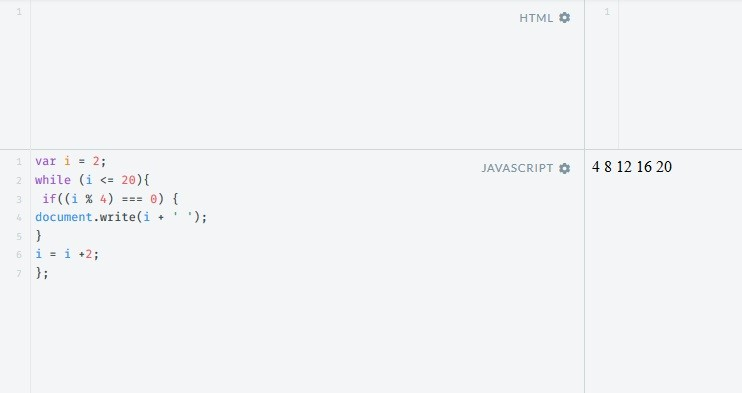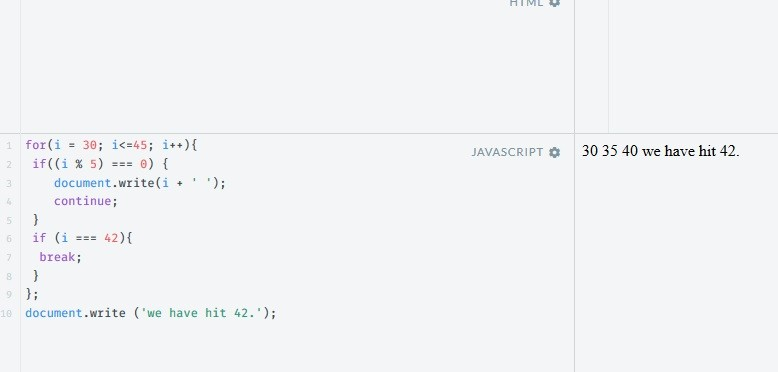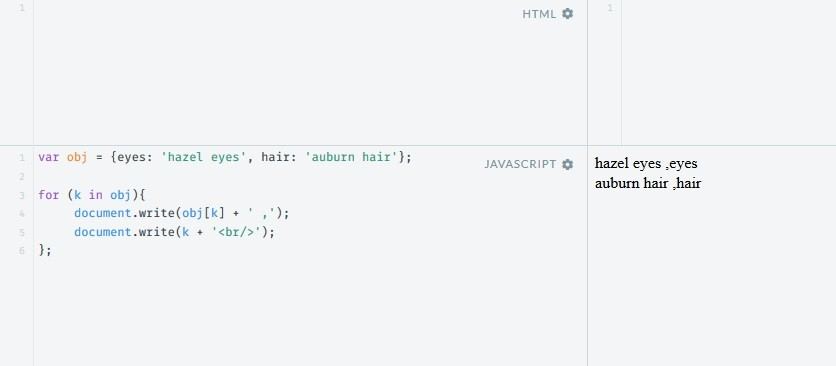Web Programming TutorialsLearn While, Do While and For Loop in JavaScript

# Learn While, Do While and For Loop in JavaScriptLooping is what we use when we want to perform an action or a set of actions an known number of times, as long as a certain condition is true. The first looping tool we’re going to look at is the while loop. The code inside the while loop’s curly brackets gets executed as long as the while condition is TRUE. Lets go over a working example.

```var i = 2;
while (i <= 20){
if((i % 4) === 0) {
document.write(i + ' ');
}
i = i +2;
};
```

Explanation: We are using a while loop to iterate over all the even numbers between 2 and 20. if a number is divisible by 4, we print it out. We started by assigning the variable i a value of 2. With each execution of the loop, we increase the value of i by 2. The loop stops running when the value of i is either equal to or bigger than 20.One of the things that distinguishes the while looping structure is that we have to create the variable to be incremented (in this case, i) before the loop, and if it fails to increment it in the loop we can get an infinite loop.

Another example of a looping structure is the do…while loop. We use this structure when we know we have to run the loop at least once.

Lets create a working example.

```Do{ var year = alert( 'Guess a year between 1950 and 1986.');
}while(year != 1979)
```

Explanation: The user is asked to guess a year. This always happens regardless of the while condition. The loop keeps running and asking the user to guess until they submit the right answer – 1979. When they do, the loop stops and the user is alerted.

Another looping structure is the for loop. It is distinguished by the fact that it is completely self-contained: i.e., creating the variable to be incremented, the condition to be checked, and action of incrementing, are all done inside this loop, and in this particular order. In this looping structure, we can use the “continue” command to immediately jump back to the beginning of the loop and increment our variable. We can use the “break” command to immediately jump out of the loop. That means we’re altogether breaking out of the looping structure, and the next command to be executed is outside of the loop.

A working example:
Lets use our example from the while loop with a slight change. Instead of printing out all the numbers divisible by 4, we’ll print out all the numbers divisible by 5 between 30 and 45. Like so:

```for(i = 30; i<=45; i++){
if((i % 5) === 0) {
document.write(i + ' ');
continue;
}
if (i === 42){
break;
}
};
document.write ('we have hit 42.');
```

Explanation: We are using a for loop to iterate over all the integers between 30 and 45 (with +1 increments). If a number happens to be divisive by 5, we print it out and then jump back to ‘i++’. This continues iterating until we get to 42, at which point we break out of the loop and print out that we have reached 42.Finally, another type of for loop is the for (k in x) loop, in which i represents the current key, and x the object to be iterated over.Working example:

```var obj = {eyes: 'hazel eyes', hair: 'auburn hair'};

for (k in obj){
document.write(obj[k] + ' ,');
document.write(k + '<br/>');
};```

Explanation: obj is an object containing key-value pairs. In this for loop, we iterate over each of the values using the current key, and print them all out, right next to their key, separated by a comma.Conclusion:
In this article we have checked out the 3 types of loop which are While, Do while and For loop in Javascript. I hope you have enjoyed this article. See you in next one.

### 6 Ways to Make Extra Money as an Introvert

21,501Fans
4,209Followers
104,000Subscribers

### 6 Ways to Make Extra Money as an Introvert

- Advertisement -